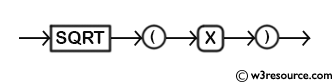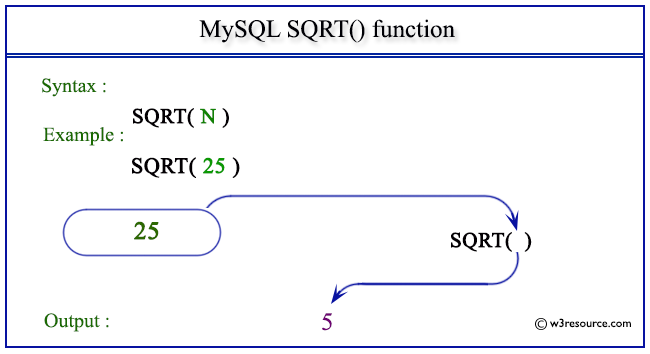# MySQL SQRT() function

## SQRT() function

MySQL SQRT() returns the square root of a non-negative number of the argument.

Syntax:

```SQRT(X)
```

Where X is a number

Syntax Diagram:MySQL Version: 5.6

Pictorial presentation of MySQL SQRT() functionExample of MySQL SQRT() function

Code:

``````SELECT SQRT(25);
```
```

Explanation

The above statement will return the square root of the given number 25.

Sample Output:

```mysql> SELECT SQRT(25);
+----------+
| SQRT(25) |
+----------+
|        5 |
+----------+
1 row in set (0.00 sec)
```

Example: SQRT() function using negative value

Code:

``````SELECT SQRT(-25);
```
```

Explanation

The above statement will return NULL because the value of the argument (-25) is negative.

Sample Output:

```mysql> SELECT SQRT(-25);
+-----------+
| SQRT(-25) |
+-----------+
|      NULL |
+-----------+
1 row in set (0.00 sec)
```

All Mathematical FunctionsPrevious: SIN()
Next: TAN()

﻿